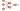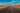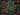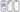Hurry Up !! Check Out Todays Deal

# Understanding Merge Sort Algorithm

Merge sort is a sorting algorithm based on "divide and conquer" technology. It is one of the most efficient classification algorithms.

In this blog, you will learn about the working principle of the merge sort algorithm, the merge sort algorithm, its time and space complexity, and its implementation in various programming languages such as C++, Python, JavaScript and Java.

## How does the merge sort algorithm work?

The operating principle of merge management is divide and conquer. Merge Sort Iteratively decompose the array into two equal sub-arrays until each sub-array contains one element. In the end, all these sub-matrices were merged times to order the resulting array.

With the help of Example, this concept can be explained more effectively. Consider an unsorted array with the following elements: {40,29, 45, 5, 11, 84, 12}.

Here, the merge sort algorithm splits the matrix into two halves, calls for the two halves, and then merges the two ordered halves.

## Space and Time Complexity of the Merge Sort Algorithm

The Merge sort algorithm can be expressed in the form of the following recurrence relation:

T(n) = 2T(n/2) + O(n)

After solving this recurrence relation using the master's theorem or recurrence tree method, you'll get the solution as O(n logn). Thus, the time complexity of the merge sort algorithm is O(n logn).

The best-case time complexity of the merge sort: O(n logn)

The average-case time complexity of the merge sort: O(n logn)

The worst-case time complexity of the merge sort: O(n logn)

The auxiliary space complexity of the merge sort algorithm is O(n) as n auxiliary space is required in the merge sort implementation.

## Merge Sorting Algorithm

Below is the Pseud code for merge sort:

``` MergeSort(arr[], left, right) if left >= right return else Find the middle index that divides the array into two halves: middle = left + (right-left)/2 Call mergeSort() for the first half: Call mergeSort(arr, left, middle) Call mergeSort() for the second half: Call mergeSort(arr, middle+1, right) Merge the two halves sorted in step 2 and 3: Call merge(arr, left, middle, right)```

### C++ Implementation of merge sort

```#include <iostream> #include <vector> #include <algorithm> #include <iterator> #include <numeric> //Function which merges the array template<class T> static void merge(T& items,auto first,auto mid,auto last) { //temp vector will hold sorted elements std::vector<typename T::value_type> temp; //reserving bytes of the memeory to avoid memory allocation temp.reserve(std::distance(first,last)); auto left = first; auto right = std::next(mid); for(auto i = first; i <= last ; ++i) { //checks if the left part come to an end of not if(left > mid){ temp.push_back(*right); right = std::next(right); } //check if the right part come to an end or not else if(right > last){ temp.push_back(*left); left = std::next(left); } //Check which elements is smaller else if(*left < *right) { temp.push_back(*left); left = std::next(left); } else{ temp.push_back(*right); right = std::next(right); } } //copies the sorted element back to the original array std::move(temp.begin(),temp.end(),first); } //Function for sorting template<class T> void mergeSort(T& items,auto first,auto last) { if(first < last) { auto mid = first;//mid iterator will point to mid element in the array std::advance(mid,std::distance(first,last)/2); //Finding the middle of array //firt half of array mergeSort(items,first,mid); //second half of array mergeSort(items,std::next(mid),last); merge(items,first,mid,last); } } //Function for printing the element template<class T> void printElement(const T& items, const std::string& heading) { std::cout << heading << std::endl; std::copy(items.begin(),items.end(), std::ostream_iterator<typename T::value_type>(std::cout," ")); std::cout << std::endl; } //Main program int main() { std::vector<int> elem({40,29, 45, 5, 11, 84, 12}); printElement(elem,"Unsorted Array:"); mergeSort(elem,elem.begin(),elem.end()); printElement(elem,"Sorted Array:"); }```

### Python Implementation of merge sort

``` def merge_sort(elem): elem_length = len(elem) if elem_length == 1: return elem mid = elem_length // 2 left = merge_sort(list[:mid]) right = merge_sort(list[mid:]) return merge(left, right) def merge(left, right): output = [] i = j = 0 while i < len(left) and j < len(right): if left[i] < right[j]: output.append(left[i]) i += 1 else: output.append(right[j]) j += 1 output.extend(left[i:]) output.extend(right[j:]) return output def main(): elem = [40,29, 45, 5, 11, 84, 12] print(elem) elem = merge_sort(elem) print(elem) ```

### JavaScript Implementation of merge sort

```<script> function merge_sort (elem) { if (elem.length === 1) { return elem } const middle = Math.floor(elem.length / 2) const left = elem.slice(0, middle) const right = elem.slice(middle) console.log(middle); return merge( merge_sort(left), merge_sort(right) ) } function merge (left, right) { let output = [] let left = 0 let right = 0 while (left < left.length && right < right.length) { if (left[left] < right[right]) { output.push(left[left]) left++ } else { output.push(right[right]) right++ } } return output.concat(left.slice(left)).concat(right.slice(right)) } const elem = [40,29, 45, 5, 11, 84, 12] console.log(merge_sort(list)); </script> ```

### Java Implementation of merge sort

```public class MergeSort { public static void main(String[] args) { int[] elem = {40,29, 45, 5, 11, 84, 12}; int[] merged = mergeSort(elem, 0, elem.length - 1); for (int val : merged) { System.out.print(val + " "); } } public static int[] merge(int[] left, int[] right) { int[] sorted = new int[left.length + right.length]; int i = 0; int j = 0; int k = 0; while (i < left.length && j < right.length) { if (left[i] < right[j]) { sorted[k] = left[i]; k++; i++; } else { sorted[k] = right[j]; k++; j++; } } if (i == left.length) { while (j < right.length) { sorted[k] = right[j]; k++; j++; } } if (j == right.length) { while (i < left.length) { sorted[k] = left[i]; k++; i++; } } return sorted; } public static int[] mergeSort(int[] elem, int left, int right) { if (left == right) { int[] br = new int; br = elem[left]; return br; } int middle = (left + right) / 2; int[] fh = mergeSort(elem, left, middle); int[] sh = mergeSort(elem, mid + 1, right); int[] output = merge(fh, sh); return output; } } ```

### Realate Post#### Understanding and building simple Neural Network using PyTorch

As the name implies, neural networks are a collection of algorithms that attempt to detect relationships in a data set using a technique that resembles the way the human brain functions.#### OpenCV - Understanding Hough Transform Using C++

In this article we will cover in-depth knowledge of what is Hough Transform, How Does it works and also how to implement it in C++#### OpenCV - Image Rotation using C++

Learn how to rotate and translate images using OpenCV. Learn about the syntax and methods for rotating and translating images.#### Javascript String Methods Cheat Sheet 2021

When programming in JavaScript, you often come across scenarios that require string manipulation. For example, when retrieving email , you may need to convert all characters to lowercase or use regular expressions to check if the entered password meets conditions.#### A Comprehansive Guid to React Native Elements

React Native Elements (RNE) are a cross-platform user interface toolkit. These elements may now be utilised on the web, and your codebase can be shared between both React Native and React web projects.